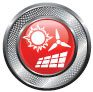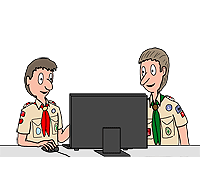# Hello, WorldNova  Award for Scouts BSA

This is a new Nova award, which was introduced in February, 2019.### This award explores the world of coding or computer programming. Once you know how to code, you can teach a computer to perform programs that help you in many ways.

1. Choose A or B or C and complete ALL the requirements.
1. Watch (not less than three hours total) computer-related shows or documentaries that involve computers, coding, and/or computer-related careers. Then do the following:
1. Make a list of at least five questions or ideas from the shows you watched.
2. Discuss two of the questions or ideas with your counselor.
2. Read (not less than three hours total) about computers, coding, and careers that involve computers. Then do the following:
1. Make a list of at least five questions or ideas from the articles you read.
2. Discuss two of the questions or ideas with your counselor.
3. Do a combination of reading and watching (not less than three hours total). Then do the following:
1. Make a list of at least five questions or ideas from the articles you read or the shows you watched.
2. Discuss two of the questions or ideas with your counselor.
2. Complete ONE merit badge from the following list. (Choose one that you have not already used for another Nova award.) Discuss with your counselor how the merit badge you earned uses coding.
 Programming Robotics Digital Technology
3. Explore different concepts in computer programming. Discuss these programming concepts with your counselor:
1. What is the difference between a local and global variable?
2. What are integer, Boolean, floating point, and character data types and what values can each of these data types hold?
3. What is a scalar variable versus an array or list variable?
4. What are these types of control flow instructions, and how do they work?
1. A While Loop
2. A Do-While Loop
3. For loop which performs a fixed number of iterations (i.e. For Loop)
4. An If statement
5. What is a function and when is it used?
6. What is a parameter to a function?
7. What is a key/value pair in a database?
8. What is a Universal Resource Locator (URL) and where is it used??
4. In a programming language of your choosing, do the following:
1. Write a program that determines if an input string of characters is a palindrome.
2. Write a program of your choice from the following list.
1. A program that calculates the sum of a set of input numbers
2. A program to report the largest and the smallest numbers from a set of input numbers.
3. A program that calculates the average of a set of input numbers.
4. A program that calculates the factorial of an input number.
5. A program that calculates the sum of all numbers from a user input starting number to a user input ending number.
These programs can be coded in a programming language such as App Lab -https://code.org/educate/applab
5. In a programming language that may be the same or different from the programing language used above, write a program selected from the following list. Work with your counselor to define the requirements — input from a user or from a file? Output to the screen or to a file? You may not use a GUI-based programming interface for this requirement, but a language like Python, Small Basic or Lisps (a family of powerful, syntax-light languages including Scheme or Common Lisp) would be appropriate.
1. A program that generates a random whole number between 1 and 10 and then asks the user to guess the number. When the guess is not correct, the program tells the user that the guess is too high or too low and then asks the user to try again. The program completes when the user correctly guesses the number.
2. A program that correctly sorts in ascending order, a sequence of at least 15 input numbers.
3. A program that accepts input, performs a calculation on the input and produces an output based on the input. It must use a function with one or more parameters to perform the calculation.
4. A program that takes a set of at least 15 characters from a user and then displays the string in reverse order.
5. A program that displays an interactive webpage that accepts user input and modifies its display based on the input (See Web Lab -https://code.org/educate/weblab for example)
6. A computer game of your choosing which uses interactive characters, requires skill and reports a winner or a loser. (See Game Lab -https://code.org/educate/gamelab for example)
7. Any other computer program agreed on by you and your counselor that uses both variables and control flow instructions.
6. For the three programs you wrote for requirements 4 and 5, explain to your counselor how each of your programs works and why you chose the particular instructions and variables that you did.
7. Do ALL of the following requirements.
1. Visit a company/school/institution where computer programs are being developed.
2. Talk to someone there about how they use coding in their work. Write down at least five questions to ask the person you visit and review the answers with your counselor
3. Discuss with your counselor how coding is being used at the destination you visited.
8. Discuss with your counselor how coding affects your everyday life, and what you have learned while working on this Nova.

### Counselor Notes:

• Requirement 1:
• Requirement 3:
Each of these concepts should be basic to computer programmers.
1. Local variable is only defined and used within one routine. A global variable is defined for the entire program
2. Integer — a variable that holds only whole numbers (e.g. 56, 7, -4, 23) Boolean — a variable that can hold only TRUE or FALSE
Floating Point — a variable that can hold any real number (e.g. 71.045)
Character variable — a variable that can hold only an alphanumeric character (e.g. "A", “G”, “&”)
3. A Scalar variable can only hold one value. An array or list variable can hold multiple values and each value can be selected using an index. (e.g. 5, 4, 10, -3 can be held in an integer array of size 4 called X. X can refer to the 3rd element of the array)
4. A while loop continuously executes the code held in the loop while a condition that is checked at the beginning of the loop is TRUE and it exits the loop when the condition checked at the beginning of the loop becomes FALSE
A Repeat loop continuously executes the code held in the loop while a condition checked at the end of the loop is TRUE and it exits the loop when the condition checked at the end of the loop becomes FALSE.
A For Loop executes the code held in the loop a fixed number of times.
An If statement executes a given computer instruction or a block of instructions if a condition is TRUE. It can also execute a different instruction or block of instructions if the condition is FALSE.
5. A function is a block of instructions which computes a result and returns the result to the calling program. A program can branch to (or call) the function and then return back to where the function was called when the function completes. A function typically computes equations needed in multiple places in the program. (e.g. a function to calculate a polynomial given an input X value.)
6. A parameter is an input to a function used in the computation of the result.
7. A Key/Value pair in database programming is a set of two linked data elements which allow the programmer to use a first key data element to find a result value based on the key (e.g. FirstName -> Joan, Lastname -> Smith)
8. A Universal Resource Locator (URL) is a set of characters which identify a resource such as a webpage or a file on the internet so a program or user may access the webpage or file.
• Requirements 4, 5 and 6:
Worksheets for use in working on these requirements: Format
Word Format PDF Format

Blanks in this worksheets table appear when we do not have a worksheet for the badge that includes these requirements.

Page updated on: April 07, 2019